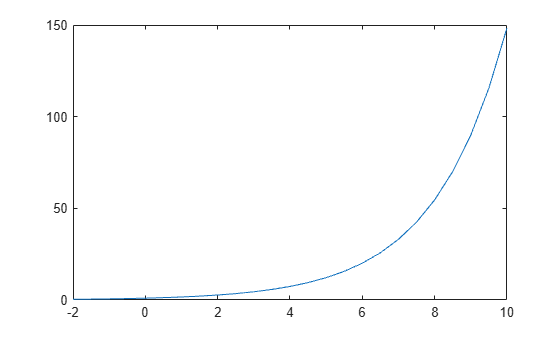# exp

## Syntax

``Y = exp(X)``

## Description

example

````Y = exp(X)` returns the exponential ex for each element in array `X`. For complex elements `z` = `x` + `iy`, it returns the complex exponential${e}^{z}={e}^{x}\left(\mathrm{cos}y+i\mathrm{sin}y\right)\text{\hspace{0.17em}}.$Use `expm` to compute a matrix exponential.```

## Examples

collapse all

Calculate the exponential of 1, which is Euler's number, e.

`exp(1)`
```ans = 2.7183 ```

Euler's identity is the equality ${e}^{i\pi }+1=0$.

Compute the value of ${e}^{i\pi }$.

`Y = exp(1i*pi)`
```Y = -1.0000 + 0.0000i ```

Plot $y={e}^{x/2}$ for `x` values in the range $\left[-2,10\right]$.

```X = -2:0.5:10; Y = exp(X/2); plot(X,Y)```## Input Arguments

collapse all

Input array, specified as a scalar, vector, matrix, or multidimensional array.

Data Types: `single` | `double`
Complex Number Support: Yes

## Output Arguments

collapse all

Exponential values, returned as a scalar, vector, matrix, or multidimensional array.

For real values of `X` in the interval (-`Inf`, `Inf`), `Y` is in the interval (`0`,`Inf`). For complex values of `X`, `Y` is complex. The data type of `Y` is the same as that of `X`.